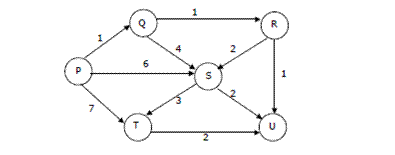GFG App
Open AppBrowser
Continue

# Data Structures and Algorithms | Set 4

Following questions have been asked in GATE CS exam.
1. Consider the following C program segment

## c

 `struct` `CellNode` `{` `  ``struct` `CelINode *leftchild;` `  ``int` `element;` `  ``struct` `CelINode *rightChild;` `}`   `int` `Dosomething(``struct` `CelINode *ptr)` `{` `    ``int` `value = 0;` `    ``if` `(ptr != NULL)` `    ``{` `      ``if` `(ptr->leftChild != NULL)` `        ``value = 1 + DoSomething(ptr->leftChild);` `      ``if` `(ptr->rightChild != NULL)` `        ``value = max(value, 1 + DoSomething(ptr->rightChild));` `    ``}` `    ``return` `(value);` `}`

The value returned by the function DoSomething when a pointer to the root of a non-empty tree is passed as argument is (GATE CS 2004)
a) The number of leaf nodes in the tree
b) The number of nodes in the tree
c) The number of internal nodes in the tree
d) The height of the tree
Explanation: DoSomething() returns max(height of left child + 1, height of left child + 1). So given that pointer to root of tree is passed to DoSomething(), it will return height of the tree. Note that this implementation follows the convention where height of a single node is 0.

2. Suppose we run Dijkstra’s single source shortest-path algorithm on the following edge weighted directed graph with vertex P as the source. In what order do the nodes get included into the set of vertices for which the shortest path distances are finalized? (GATE CS 2004)
a) P, Q, R, S, T, U
b) P, Q, R, U, S, T
c) P, Q, R, U, T, S
d) P, Q, T, R, U, S3. Suppose each set is represented as a linked list with elements in arbitrary order. Which of the operations among union, intersection, membership, cardinality will be the slowest? (GATE CS 2004)
a) union only
b) intersection, membership
c) membership, cardinality
d) union, intersection
Cardinality and membership are definitely not the slowest one. For cardinality, just count the number of nodes in a list. For membership, just traverse the list and look for a match
For getting intersection of L1 and L2, search for each element of L1 in L2 and print the elements we find in L2.
There can be many ways for getting union of L1 and L2. One of them is as follows
a) Print all the nodes of L1 and print only those which are not present in L2.
b) Print nodes of L2.

4. The time complexity of the following C function is (assume n > 0 (GATE CS 2004)

## c

 `int` `recursive (mt n)` `{` `   ``if` `(n == 1)` `     ``return` `(1);` `   ``else` `     ``return` `(recursive (n-1) + recursive (n-1));` `}`

a) 0(n)
b) 0(nlogn)
c) 0(n^2)
d) 0(2^n)
Explanation:
Recursive expression for the above program will be.

```T(n) = 2T(n-1) + c
T(1) = c1.```

Let us solve it.

```T(n) = 2(2T(n-2) + c) + c        = 4T(n-2) + 3c
T(n) = 8T(n-3) + 6c + c          =  8T(n-3) + 7c
T(n) = 16T(n-4) + 14c + c        =  16T(n-4) + 15c
...................................................
...................................................
T(n) = (2^(n-1))T(1) +  (2^(n-1) - 1)c
T(n) = O(2^n)```